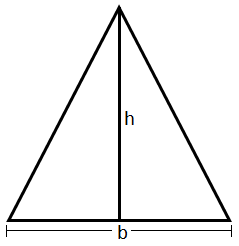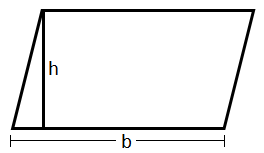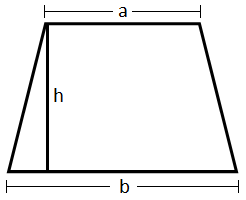# What is the area of triangle, parallelogram, and trapezium?

Area\ of\ triangle\ =\ \frac{1}{2} \ \times \ Base\ \times \ Height\\
\\
\\
Area\ of\ triangle\ =\ \frac{1}{2} \ \times \ b\ \times \ h
\end{array}1)  Area of triangle = 21 × Base × Height
Area of triangle = 21 × b × h2)   Area of parallelogram = Base × Height

Area of parallelogram = b × h

Area\ of\ Trapezium\ =\ \frac{1}{2} \ \times \ ( Sum\ of\ parallel\ sides) \ \times \ Distance\ between\ parallel\ sides\\
\\
\\
Area\ of\ Trapezium\ =\ \frac{1}{2} \ \times \ ( a\ +\ b) \ \times \ h
\end{array}3)  Area of Trapezium = 21 × (Sum of parallel sides) × Distance between parallel sides
Area of Trapezium = 21 × (a + b) × h

Updated on: 10-Oct-2022

26 Views